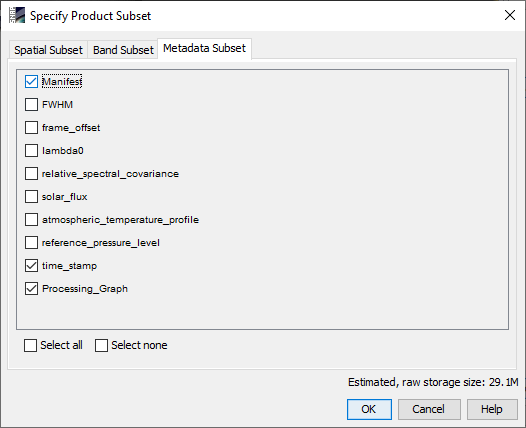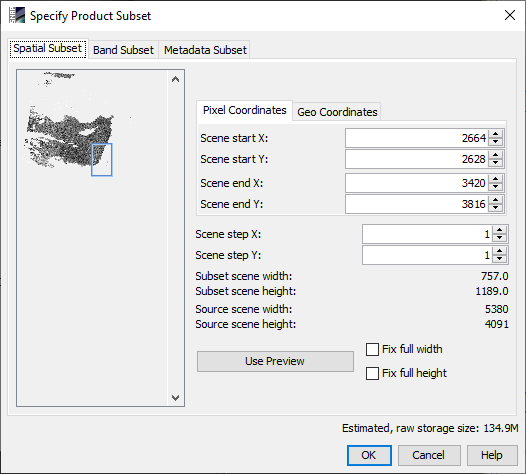# SNAP vs MATLAB - values not the same

Hello everyone,

The steps I make to export the S3B L2 (WFR) image to GeoTIFF from SNAP software:

1. RASTER -> Geometric Operations -> Reprojection -> WGS 84 with reproject tie points grid and preserve resolution.
2. RASTER -> Subset -> choose what I want to keep.
3. File -> Export -> GeoTIFF.

Images of the setup is included in this topic. (if need more details, tell me)SUBSET~1.HDR (772 Bytes)

After exporting to GeoTIFF, I opened the file in MATLAB using “imread” function and checked if the values are the same as in SNAP before continuing in my process work.

At the bottom line, I checked at the level pixel and found the reflectance bands are the same values in MATLAB and in SNAP - that’s good.
On the other hand, the values of calculated indicators (CHL_NN, CHL_OC4ME, etc) are different between SNAP and MATLAB !?!?

I did the comparison using the same GeoTIFF file I export.

For example: (included images:)

1. CHL_NN: a value of 10.94 mgm-3 is equal to 1.039 in MATLAB, and a value of 1 mgm-3 is equal to 0 in MATLAB.

2. TSM: a value of 13.61 g*m-3 is equal to 1.134 in MATLAB.

I also check with ENVI and the values are the the same as Matlab!

So, I suppose that SNAP s3tbx have a different approach to show the pixel info than ENVI and MATLAB.

I would like to know how to show the values of the calculated indicators in MATLAB like in SNAP (that take into account the unit of the indicators maybe ?)

Maybe there is a different way to export, so MATLAB will show the same values ?
Or, maybe there is a scaling method to do, but one that is not static because I tried and see that the values are different in every pixel… so the scaling work for the current pixel scaled but less for the others.

Need some helpThanks,
Natan Krief

If I understand, you are creating geotiffs in SNAP and comparing values seen when the geotiff is leaded in Matlab/ArcGIS. Do you get any warning messages in Matlab/ArcGIS when you load the geotiff files? Did you try loading the geotiff in SNAP?

In the past I have used SeaDAS 7/BEAM to create geotiffs for GIS users and encountered problems with GIS software that didn’t support some of the datatypes used by BEAM. If your tools support NetCDF4-CF, that format has more complete metadata. Geotiff is not a formal standard so apps may not handle all geotiffs the same way.

If you have GDAL, you can use the `gdalinfo` tool to examine the geotiff files. Look for differences in metadata between files that import correctly and those that give different values.

No warnings.

Yes. That’s what I said and that’s why I think that the problem is MATLAB or ENVI that don’t know how to show the value as showed in SNAP.

I used SNAP s3tlbx to export to GeoTiff as mentioned earlier in my Topic.
I didn’t want to use Matlab with nc format file because I already make a lot of functions that will work with GeoTiff format.
If there is no solution maybe I’ll use the nc files directly in Matlab or SNAP but then I’ll need to do a lot of rearrangement in all my Matlab functions…So I’ll prefer not to…

In the mean time,
The xml file of the S3 L2 original image (nc files - before the reprojection, subset an export) I used:
xfdumanifest.xml (171.9 KB)

The HDR file by SNAP, after reprojecting, subseting and exporting the original image to GeoTiff :
SUBSET~1.HDR (772 Bytes)

The GeoTiff info from SNAP + info about CHL_NN for example (in the Information section):

And here is the GeoTiff info from MATLAB using the function “geotiffinfo”:

I didn’t see differences yet, maybe you could check and told me if there is differences?

Again, for the reflectance bands there are no differences in the values!
That’s why I’m pretty sure that SNAP is doing something with the raw values of CHL_NN, CHL_OC4ME, TSM, etc, before showing them in the Pixel Info section in SNAP and that’s why there are differences between SNAP and MATLAB/ENVI.

So, I want to know what SNAP is doing (if my supositions are right) to reproduce the same in MATLAB and then get the same value in MATLAB and work from here…

…still need helpCheers,
N.K

In snap, the CHL_NN metadata indicates the values are “log scaled”. I don’t think GeoTiff tags support log scaling. Are the problem bands the ones that use log scaling?

Using R:

``````> log10(10.94)  ## CHL_NN
 1.039017
``````

log10(13.61)
 1.133858

In my experience, if this is something you plan to do often, then investing the time to use NetCDF4-CF is well worth the savings in having to manually reconcile issues like this and finding a way to make sure the missing metadata aren’t lost when you go back to the geotiff files in a year.

1 Like

I just did 10^ of the data that was needed to scale.

Work like a charm!

Thanks gnwiii !!